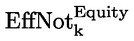In force

Version from: 01/01/2023
Amendments
Search within this legal act

### Article 280d - Equity risk category add-on

Article 280d

1.

For the purposes of paragraph 2, institutions shall establish the relevant equity reference entities of the netting set in accordance with the following:

(a)

there shall be one equity reference entity for each issuer of a reference equity instrument that underlies a single-name transaction allocated to the equity risk category; single-name transactions shall be assigned to the same equity reference entity only where the underlying reference equity instrument of those transactions is issued by the same issuer;

(b)

there shall be one equity reference entity for each group of reference equity instruments or single-name equity derivatives that underlie a multi-name transaction allocated to the equity risk category; multi-names transactions shall be assigned to the same equity reference entity only where the group of underlying reference equity instruments or single-name equity derivatives of those transactions, as applicable, has the same constituents.

2.

For the purposes of Article 278, institutions shall calculate the equity risk category add-on for a given netting set as follows:where:

 j = the index that denotes all the equity risk hedging sets established in accordance with point (d) of Article 277a(1) and Article 277a(2) for the netting set; and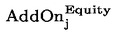= the equity risk category add-on for hedging set j calculated in accordance with paragraph 3.
3.

Institutions shall calculate the equity risk category add-on for hedging set j as follows: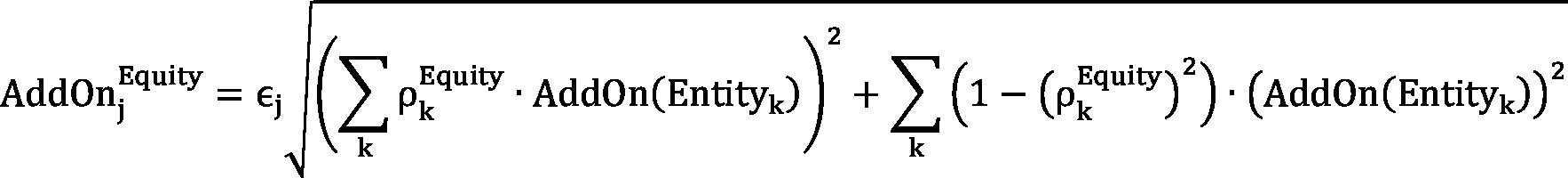where: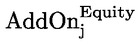= the equity risk category add-on for hedging set j;
 єj = the hedging set supervisory factor coefficient of hedging set j determined in accordance with Article 280;
 k = the index that denotes the equity reference entities of the netting set established in accordance with paragraph 1;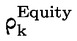= the correlation factor of the equity reference entity k; where the equity reference entity k has been established in accordance with point (a) of paragraph 1,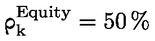; where the equity reference entity k has been established in accordance with point (b) of paragraph 1,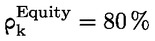; and
 AddOn(Entityk) = the add-on for the equity reference entity k determined in accordance with paragraph 4.
4.

Institutions shall calculate the add-on for the equity reference entity k as follows: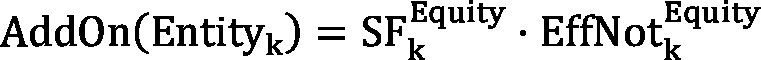where: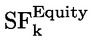= the supervisory factor applicable to the equity reference entity k; where the equity reference entity k has been established in accordance with point (a) of paragraph 1,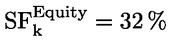; where the equity reference entity k has been established in accordance with point (b) of paragraph 1,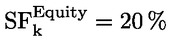; and